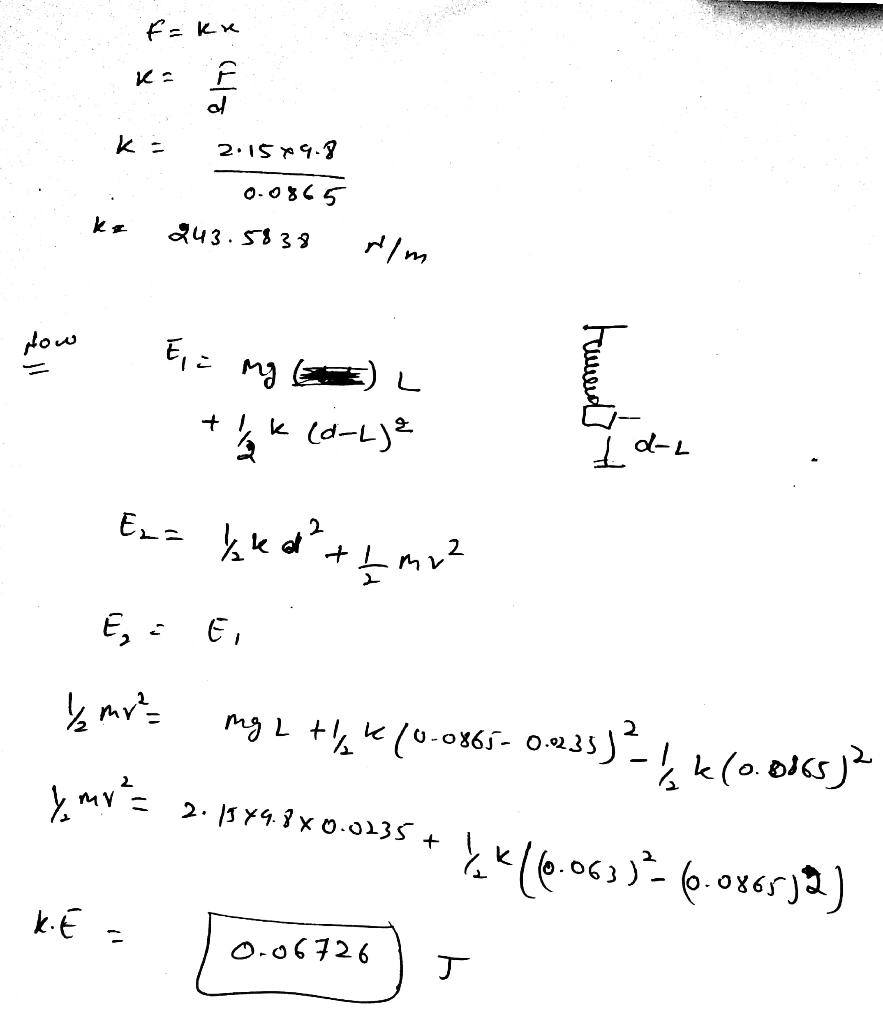Homework Help Question & Answers

An ideal spring hangs from the ceiling. A 2.15 kg mass is hung from the spring, stretching the sp...

An ideal spring hangs from the ceiling. A 2.15 kg mass is hung from the spring, stretching the spring a distance d = 0.0865 m from its original length when it reaches equilibrium. The mass is then lifted up a distance L = 0.0235 m from the equilibrium position and released. What is the kinetic energy of the mass at the instant it passes back through the equilibrium position?Add Answer of: An ideal spring hangs from the ceiling. A 2.15 kg mass is hung from the spring, stretching the sp...
More Homework Help Questions Additional questions in this topic.

• The demand for ceiling fans can be modeled below as D(p) 25.52(0.996P) thousand ceiling fans where p is the price (in dollars) of a ceiling fan (a) According to the model, is there a price above whic...

Need Online Homework Help?

Get FREE EXPERT Answers
WITHIN MINUTES
Related Questions